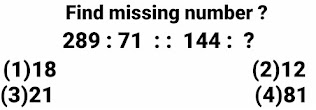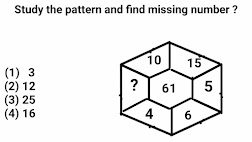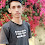# Ten Important Reasoning questions with answers for competitive exams

## Problem # 1SSC CGL Reasoning
This reasoning problem consists of two figures and every figure have five numbers associated to it . So the solution of this problem is to find the value of question mark using other four numbers associated to it .
But the major problem is how to utilised these four numbers to get the value of this question mark?
Now watch carefully the 1st figures . Since this figure have some values of middle numbers.
Now we have to find or search the  formula for these four numbers in 1st figure to utilised them in any possible way to get middle or central number.
The same formula will be applicable to third figure to find out the value of question mark.
Formula :- The difference of  product of both the numbers in upper row and product of both the numbers in lower row is equal to middle number in every figure.
( 11 × 12 ) - ( 6 × 9 ) = 132 - 54 = 78 (Middle number in 1st figure )
( 14 × 10 ) - ( 7 × 8 ) = 140 - 56 = 84 (Middle number in 2nd figure)
Option (1)84 is correct option

## Problem # 2SSC CGL Reasoning
This reasoning problem consists of only one figure and this figure have eight numbers associated to it . So the solution of this problem is to find the value of question mark using other numbers associated to it .
But the major problem is how to utilised these four numbers to get the value of this question mark?
Formula :- Sum of  twice of any number in the inner circle and its adjoining number taken in clockwise order is equal to number in the outer circle.
( 3 × 2 ) + 4 (number when moving from  3 clockwise ) = 6 + 4 = 10 ( Number in the outer circle )
( 4 × 2 ) + 6 (number when moving from 3 clockwise ) = 8 + 6 = 14 ( Number in the outer circle )

( 6 × 2 ) + 8 (number when moving from  3 clockwise ) = 12 + 8 = 20 ( Number in the outer circle )

( 8 × 2 ) + 3  (number when moving from 3 clockwise ) = 16 + 3 = 19 ( Number in the outer circle )
Option (1)19 is correct option

## Problem # 3SSC CGL Reasoning
This reasoning problem consists of three figures and every figure have three numbers associated to it . So the solution of this problem is to find the value of question mark using other two numbers associated to it .
But the problem is how to utilised these two numbers to get the value of this question mark?
Now we have to find or search the  formula for these two numbers in each figure to utilised them in any possible way to get third number.
The same formula will be applicable to third figure to find out the value of question mark.
Formula :- The sum of  two numbers which are on the perpendicular lines is equal to third number of triangle in every figure.
( 13 + 19 ) ÷ 8 = 32 ÷ 8 = 4 ( 3rd number in 1st triangle )
( 71 + 9 ) ÷ 8 = 80 ÷ 8 =  10 ( 3rd number in 1st triangle )
( 128 + 32 ) ÷ 8 = 160  ÷ 8 = 20 ( 3rd number in 1st triangle )
Option (2)20  is correct option

## Problem # 4SSC CGL Reasoning
This reasoning problem consists of three figures and every figure have five numbers associated to it . So the solution of this problem is to find the value of question mark using other four numbers associated to it .
But the biggest problem is how to utilised these four numbers to get the value of this question mark?
Now watch carefully the 1st two figures . since these figures have some values of middle numbers.
Now we have to find or search the  formula for these four numbers in each figure to utilised them in any possible way to get middle or central number. The same formula will be applicable to third figure to find out the value of question mark.

Formula :- The sum of  product of left & right and sum of lower and upper numbers in each figure is equal to middle number in every figure.
( 6 × 7 ) + ( 8 + 4 ) = 42 + 12 = 54 ( Middle number in 1st figure ) .
( 8 × 4 ) + ( 12 + 7 ) = 32 + 19 = 51 ( Middle number in 2nd figure ) .
( 9 × 5 ) + ( 14 + 9 ) = 45 + 23 = 68 ( Middle number in 3rd figure ) .
Option (2)68 is correct option

## Problem # 5SSC CGL Reasoning

This reasoning problem consists of three figures and every figure have six numbers associated to it . So the solution of this problem is to find the value of question mark using other five numbers associated to it .
But the biggest problem is how to utilised these four numbers to get the value of this question mark?
Now watch carefully the 1st two figures . Since these figures have some values in middle numbers.
Now we have to find or search the  formula for these four numbers in each figure to utilised them in any possible way to get middle or central number.
The same formula will be applicable to third figure to find out the value of question mark.
Formula :- The product of top three numbers in any figure is equal to product of other three numbers in every figure.
(4 × 3 × 5 ) = 60 =  ( 20 × 3 × 1 )  ( Multiplication of top three numbers is equal to multiplication  of bottom three numbers ) .
(2 × 4 × 5 )  = 40 =  ( 10 × 2 × 2 )  ( Multiplication of top three numbers is equal to multiplication  of bottom three numbers ) .
( 8 × 6 × 1 ) = 48 =  ( ? × 3 × 4 ) =  Multiplication of top three numbers is equal to multiplication  of bottom three numbers ) .
? = 48 ÷  12
? = 4
Option (1)4 is correct option

##SSC CGL Reasoning

In these types of reasoning problems 2nd number will be obtained from 1st number either by using one mathematical operation/ set of mathematical operations or by using any mathematical operation/ set of mathematical operations to individual digits of 1st number. Same  mathematical operation/ set of mathematical operations  or formula will be used in 3rd number to get fourth number.
Formula :-  (1st digit  ×2nd digit÷  middle digit  = 2nd number
( 5  × 6  ) ÷ 2 = 30 ÷ 2 = 15
( 8  ×  3 ) ÷ 3 = 24 ÷ 3 = 8
Option (1)8 is correct option

##SSC CGL Reasoning

In these types of reasoning problems 2nd number will be obtained from 1st number either by using one mathematical operation/ set of mathematical operations or by using any mathematical operation/ set of mathematical operations to individual digits of 1st number. Same  mathematical operation/ set of mathematical operations  or formula will be used in 3rd number to get fourth number.
Formula :-  Reversing the digits of square root of 1st number = 2nd number
(√289 )= 17  ⇔ 71 (After reversing the order of digits )
√ (144 )= 12 ⇔ 21 (After reversing the order of digits )
Option (3)21 is correct option

##SSC CGL Reasoning

In these type of reasoning problems 2nd number will be obtained from 1st number either by using one mathematical operation/ set of mathematical operations or by using any mathematical operation/ set of mathematical operations to individual digits of 1st number. Same  mathematical operation/ set of mathematical operations  or formula will be used in 3rd number to get fourth number.

Formula :-  1st digit ^  2nd digit =  2nd number

Taking 1st digit as base and and digit as power then after expansion we have

2⁶ = 2 × 2 × 2 × 2 × 2 × 2 = 64

3⁵ = 3 × 3 × 3 × 3 × 3 = 243

Option (1)243 is correct option

## Problem # 9SSC CGL Reasoning

This reasoning problem consists of only one figure and this figure have eight numbers associated to it . So the solution of this problem is to find the value of question mark using other numbers associated to it .
But the major problem is how to utilised these four numbers to get the value of this question mark?

Formula :- ( Any number   × Opposite of number already chosen ) + 1 = 61

(10 × 6) +1 = 60 +1 = 61(Middle number)

(15 × 4) +1 = 60 +1 = 61(Middle number)

(5 × ? ) +1 = 60 +1 = 61(Middle number)

(5 × ? ) +1 = 61

(5 × ? )  = 60

?   = 60 ÷ 5

?  = 12

Option (2)12 is correct option

## Problem # 10SSC CGL Reasoning
This reasoning problem consists of only one figure and this figure have eight numbers associated to it . So the solution of this problem is to find the value of question mark using other numbers associated to it .

But the major problem is how to utilised these four numbers to get the value of this question mark?

Formula :- ( Any number   + Opposite of number already chosen ) + 13 = 71

(23 + 35 ) + 13 = 58 + 13 = 71(Middle number)

(41 + 17 ) + 13 = 58 + 13 = 71(Middle number)

(46 + ? ) + 13 =   71(Middle number)

46 + ?  =   71 - 13

46 + ? =   58

? = 58 - 46

? = 12

Option (2)12 is correct option.
In this post I discussed Ten Tricky logical reasoning questions+answers , Latest reasoning questions with answers  . Comment your valuable suggestion for further improvement.

1.2.